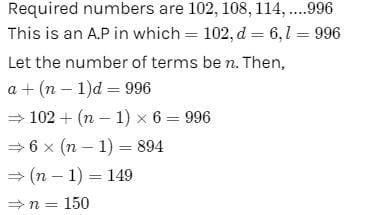# Test: Algebra

## 10 Questions MCQ Test NCERT Mathematics for CAT Preparation | Test: Algebra

Description
Attempt Test: Algebra | 10 questions in 10 minutes | Mock test for Class 6 preparation | Free important questions MCQ to study NCERT Mathematics for CAT Preparation for Class 6 Exam | Download free PDF with solutions
QUESTION: 1

Solution:
QUESTION: 2

Solution:
QUESTION: 3

### Number of matchsticks required to make a pattern of “E”

Solution:

Number of matchsticks required to make one= 5
∴ Number of matchsticks required to make a pattern of letter E as= 5n

QUESTION: 4

A basket has x mangoes, how many mangoes are there in 5 baskets?

Solution:
QUESTION: 5

Perimeter of the square, whose each side is ‘n’ cm is

Solution:
QUESTION: 6

Diameter of circle whose radius is ‘r’ is

Solution:

Diameter is twice the radius, so in this case it'll be 2r

QUESTION: 7

The number of 3-digit numbers divisible by 6, is …………...

Solution:QUESTION: 8

The side of an equilateral triangle is shown by l. Express the perimeter of the equilateral triangle using l.

Solution:
QUESTION: 9

Give expression for 25 added to r.

Solution:
QUESTION: 10

Which out of the following are expressions with numbers only?

Solution:Use Code STAYHOME200 and get INR 200 additional OFF Use Coupon Code